Next: Worked example 6.6: 2-dimensional Up: Conservation of momentum Previous: Worked example 6.4: Bullet

## Worked example 6.5: Elastic collision

Question: An object of mass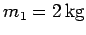, moving with velocity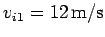, collides head-on with a stationary object whose mass is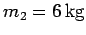. Given that the collision is elastic, what are the final velocities of the two objects. Neglect friction.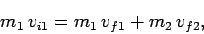where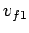and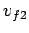are the final velocities of the first and second objects, respectively. Since the collision is elastic, the total kinetic energy must be the same before and after the collision. Hence,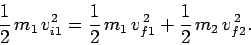Let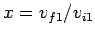and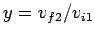. Noting that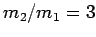, the above two equations reduce to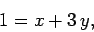and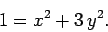Eliminatingbetween the previous two expressions, we obtain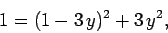or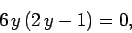which has the non-trivial solution. The corresponding solution foris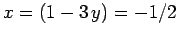.

It follows that the final velocity of the first object is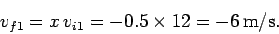The minus sign indicates that this object reverses direction as a result of the collision. Likewise, the final velocity of the second object is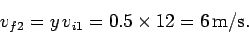Next: Worked example 6.6: 2-dimensional Up: Conservation of momentum Previous: Worked example 6.4: Bullet
Richard Fitzpatrick 2006-02-02